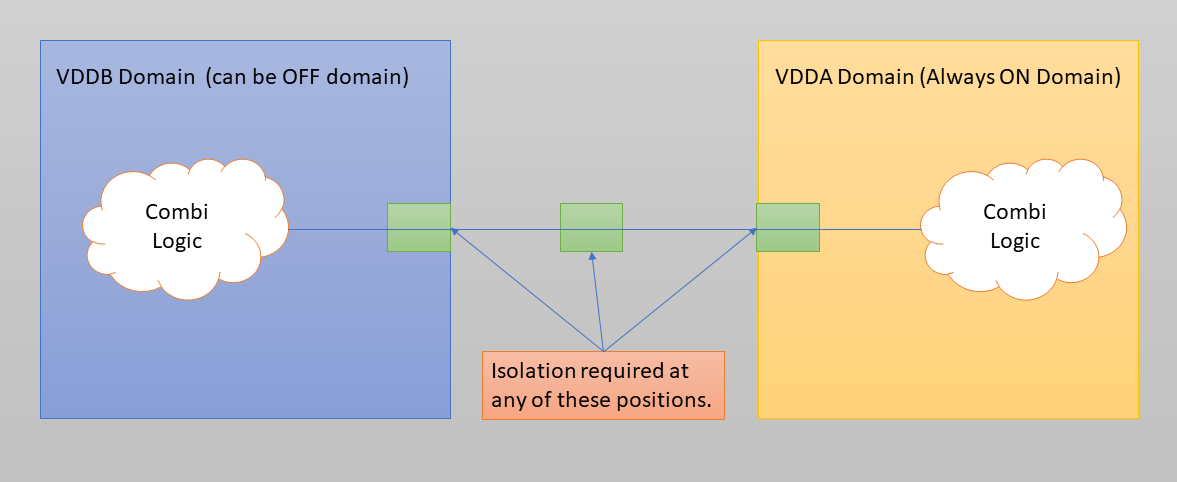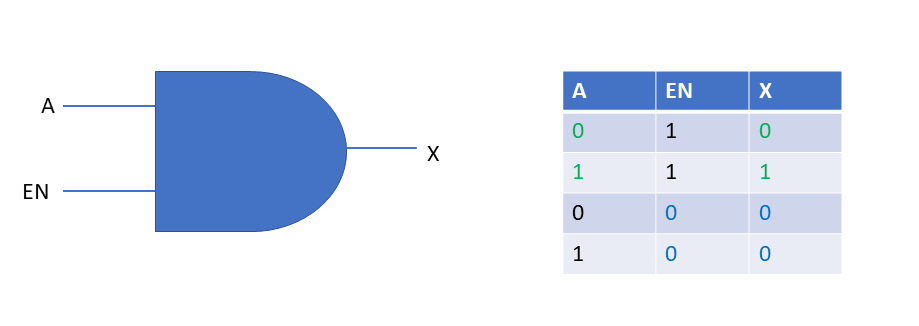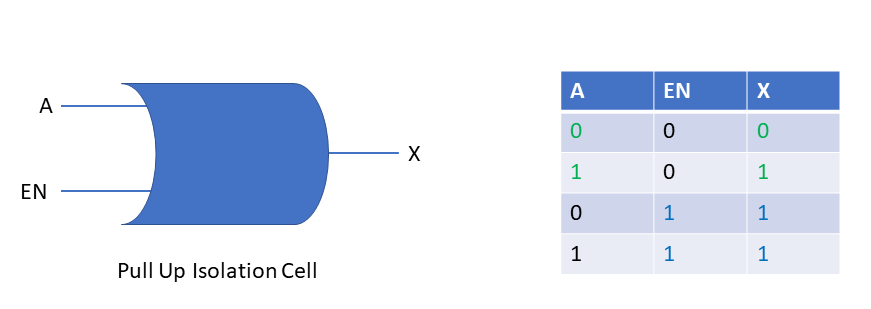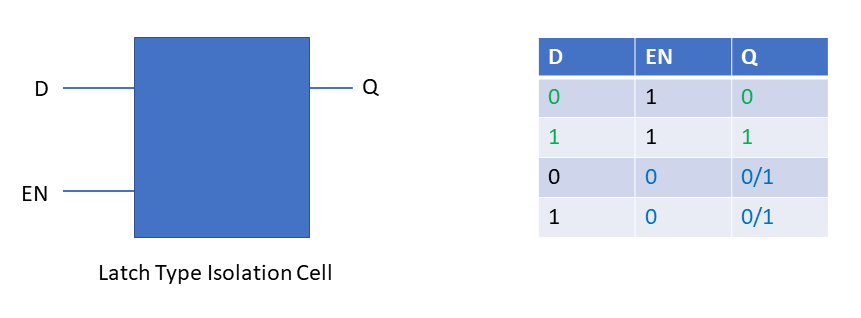# Understanding Isolation Cells in UPF CLP | Requirement Of Isolation Cells in VLSI Low Power Check

In this post, I am going to discuss about isolation cell in detail. Isolation cells are indispensable part of a multi voltage design in current vlsi world.

### What is an Isolation Cell?

Isolation cell is a basic logic gate which is used to pull-up (logic 1)  or pull-down ( logic 0 )  a logic node when the node is supposed to get invalid logic (unknown voltage/ intermediate voltage) . This invalid logic may happen when the design is having more than one voltage and the voltages are kept on all the time. Let’s say voltage domain VDDA is on all the time after the initial turning on a chip. The other voltage domain VDDB may switch off in between depending on some condition.  When the VDDB domain is switched the the output of all the logic gets associated with VDDB domain would be at logic x or unknown state (OFF state). This VDDB logic gates may be driving some VDDA logic and the VDDA logic is expecting logic value as it’s not in OFF state. But as VDDB logic gates are driving logic x, the functionality gets corrupted. To mitigate this, we need isolation cell which will drive the required logic 0, logic 1 or latch value to VDDA domain.### Isolation Cell Types

#### Pull Down/ Clamp to Zero Isolation Cell:

The pull-down isolation cells are simple AND gates. For an AND gate, if you make one input zero then the output would be at logic zero. So, AND gate is placed in between VDDB logic and VDDA logic . The other input of AND gate is connected to control input that goes to zero whenever there is OFF (VDDB) to ON (VDDA) type scenario happens. From the below, we can see when isolation control input EN is at logic 1, output is same as input and functionality is intact. But when EN is zero isolation cell output is at logic zero and it doesn’t matted what the input is.There is another special type pull-down isolation cell is present which is a NOR gate with inverted logic input A. The EN input is not inverted. The output can be written as X=A.EN’ . This is useful in cases where the isolation cell is placed in OFF domain (VDDB) itself. It doesn’t require any power supply to pull down the node as power is off in off domain.

#### Pull UP/ Clamp to One Isolation Cell

The pull up isolation cells are simple OR gates. When isolation is not required isolation control signal is at logic 0, and output is same as input. But when isolation is required with clamping to logic 1 , the control input EN goes to logic 1 and the output is pulled to logic 1 irrespective of logic input A.#### Latch Type Isolation Cell

Latch type isolation cells are simple D-latch. These isolation cells are used when the clamp value is not certain and latched value is fed to VDDA domain. This works with the same principal. When the D-latch control input EN is at logic 1, the input is same as output. But when the latch control input goes to logic zero, the captured value is fed to the output. The output  can be at logic 0 or 1, depending at captured value. But the captured value won’t change even if the input D value changes.#### Important Points

• Latch type isolation cells should have dual supply. This is required because to hold the value it needs supply.
• Pull up isolation cells can have  dual supply ( two supply). These can be kept in OFF domain even if the off domain supply is not available.
• Similarly, Pull down AND type isolation can be of dual supply type. These can be placed in off domain with on supply.
• For NOR type pull down isolation cell only single supply is required.

Keywords

cell isolation,clp in vlsi,clp vlsi,iso cells,isolation cell,isolation cell in vlsi,isolation cell vlsi,isolation cell working,isolation cells,isolation cells in vlsi,isolation cells vlsi,isolation in vlsi,types of isolation cells in vlsi,what are isolation cells in vlsi,what is isolation cell in vlsi,